﻿ On a Class of P-Kenmotsu Manifolds Admitting Weyl-projective Curvature Tensor of Type (1, 3)Publications are Open
Access in this journal
Article Versions
Export Article
• Normal Style
• MLA Style
• APA Style
• Chicago Style
Research Article
Open Access Peer-reviewed

### On a Class of P-Kenmotsu Manifolds Admitting Weyl-projective Curvature Tensor of Type (1, 3)

K. L. Sai Prasad , S. Sunitha Devi, G. V. S. R. Deekshitulu
Turkish Journal of Analysis and Number Theory. 2018, 6(6), 155-158. DOI: 10.12691/tjant-6-6-2
Received September 11, 2018; Revised October 23, 2018; Accepted November 24, 2018

### Abstract

We study a class of para-Kenmotsu manifolds admitting Weyl-projective curvature tensor of type (1, 3). At the end, it is shown that an n-dimensional (n > 2) P-Kenmotsu manifold is Ricci semisymmetric if and only if it is an Einstein manifold.

### 1. Introduction

In 1, 2, Sato introduced the notions of an almost para contact Riemannian manifold. In 1977, Adati and Matsumoto defined para-Sasakian and special para-Sasakian manifolds, which are regarded as a special kind of an almost contact Riemannian manifolds 3. Para-Sasakian manifolds have been studied by Adati and Miyazawa 4, De and Avijit 5, Matsumoto, Ianus and Mihai 6 and many others. Before Sato, Kenmotsu defined a class of almost contact Riemannian manifolds 7. In 1995, Sinha and Sai Prasad defined a class of almost para contact metric manifolds namely para-Kenmotsu (briefly P-Kenmotsu) and special para-Kenmotsu (briefly SP-Kenmotsu) manifolds 8.

In 1970, Pokhariyal and Mishra introduced new tensor fields, called W2 and E tensor fields, on a Riemannian manifold 9. Later, in 10, Pokhariyal studied some of the properties of these tensor fields on a Sasakian manifold. In 1986, Matsumoto, Ianus and Mihai have extended these concepts to almost para-contact structures and studied para-Sasakian manifolds admitting these tensor fields 6. These results were further generalised by De and Sarkar, in 5. Motivated by these studies, in 2015, Sai Prasad and Satyanarayana studied W2-tensor field in an SP-Kenmotsu manifold 11. In the present work, we investigate a class of para-Kenmotsu manifolds admitting Weyl-projective curvature tensor W2 of type (1, 3). The present work is organised as follows: Section 2 is equipped with some prerequisites about P-Kenmotsu manifolds. In Section 3, we define W2-recurrent and semisymmetric para-Kenmotsu manifolds and shown that W2-recurrent para-Kenmotsu manifold is a semisymmetric manifold. Further, it is shown that the curvature of W2-semisymmetric para-Kenmotsu manifold is constant and hence we establish that a W2-recurrent para-Kenmotsu manifold is an SP-Kenmotsu manifold. Section 4 is devoted to study Ricci semisymmetric P-Kenmotsu manifold.

### 2. Preliminaries

Let Mn be an n-dimensional differentiable manifold equipped with structure tensors (, ξ, η) where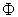is a tensor of type (1, 1), ξ is a vector field, η is a 1-form such that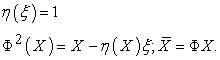(2.1)

Then the manifold Mn is called an almost para-contact manifold.

Let g be a Riemannian metric such that, for all vector fieldsandon Mn(2.2)

Then the manifold Mn 1 is said to admit an almost para-contact Riemannian structureIn addition, ifsatisfies the conditions(2.3)

then Mn is called para-Kenmotsu manifold or briefly a P-Kenmotsu manifold 8.

A P-Kenmotsu manifold admitting a 1-form η satisfying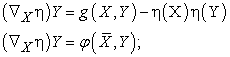(2.4)

whereis an associate ofis called special para-Kenmotsu manifold or briefly SP- Kenmotsu manifold 8.

Let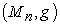be an n-dimensional,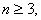differentiable manifold of classand let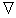be its Levi-Civita connection. Then the Riemannian Christoffel curvature tensor R of type (1, 3) is given by: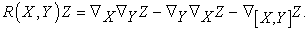(2.5)

The Ricci operator S and the (0, 2)- tensor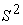are defined by(2.6)

and(2.7)

It is known 8 that in a P-Kenmotsu manifold the following relations hold: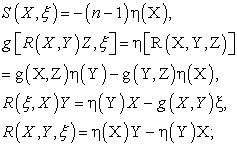(2.8)

when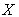is orthogonal to 𝛏.

An n-dimensional (n > 2) Riemannian manifold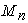is said to be Einstein manifold if the Ricci curvature tensor S(X, Y) of the Levi-Civita connection satisfies the condition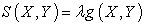(2.9)

whereis a constant.

### 3.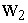- Recurrent P-Kenmotsu Manifolds

The Weyl-projective curvature tensorof type (1, 3) of a Riemannian manifoldwith respect to Riemannian connection is given by 9: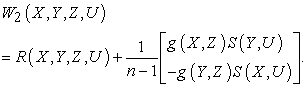(3.1)

Now, we define a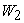-semisymmetric para-Kenmotsu manifold as:

Definition 3.1: An n-dimensional para-Kenmotsu manifold is called-semisymmetric if its-curvature tensor satisfies the condition(3.2)

whereis considered to be a derivation of the tensor algebra at each point of the manifold for tangent vectorsand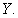It can be easily shown that on a P-Kenmotsu manifold the-curvature tensor satisfies the condition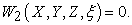(3.3)

Further, we define a-recurrent para-Kenmotsu manifold as:

Definition 3.2: An n-dimensional para-Kenmotsu manifold with respect to the Levi-Civita connection is called-recurrent manifold if its-curvature tensor satisfies the condition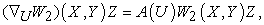(3.4)

where A is some non-zero 1-form.

Now, let us establish a relation between-recurrent and-semisymmetric para-Kenmotsu manifolds.

For that, let us suppose that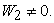Now, we define a function by(3.5)

Using the fact that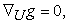from (3.5) we getSincewe have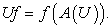(3.6)

Then, from (3.6), we get(3.7)

and hence, we have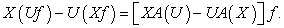(3.8)

Therefore,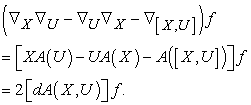(3.9)

Since the left hand side of (3.9) is zero and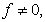we deduce thatand it shows that the 1-form A is closed.

Then from (3.4), we get that(3.10)

and hence, we get that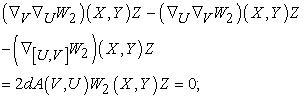(3.11)

i.e.,where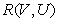is considered to be a derivation of tensor algebra at each point of the manifold for the tangent vectors V and U.

This shows that a-recurrent P-Kenmotsu manifold is-semisymmetric and hence we state that:

Theorem 3.1: A-recurrent para-Kenmotsu manifold is-semisymmetric.

Further we determine the curvature value of-semisymmetric P-Kenmotsu manifold.

From (3.2), we have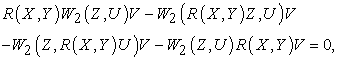(3.12)

which implies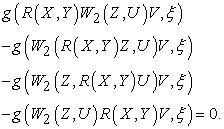(3.13)

By puttingin the above equation, we get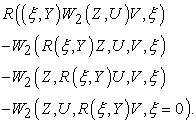(3.14)

Now, by using (2.8) and (3.3), the above equation reduces to:(3.15)

Again on using (3.3), we get thatTherefore, from (3.1) we have(3.16)

On contracting the above equation, we get(3.17)

Then, from equations (3.16) and (3.17), we have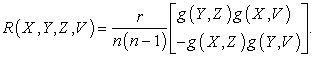(3.18)

This shows that the curvature of-semisymmetric P-Kenmotsu manifold is constant.

As it is known 8 that a P-Kenmotsu manifold with constant curvature is an SP-Kenmotsu manifold and using the above shown result, we state that:

Theorem 3.2: A-semisymmetric P-Kenmotsu manifold is an SP-Kenmotsu manifold.

Therefore, form theorems (3.1) and (3.2), we have the following result:

Theorem 3.3: A-recurrent P-Kenmotsu manifold is an SP-Kenmotsu manifold.

### 4. Ricci Semisymmetric Para-Kenmotsu Manifolds

Definition 4.1: An n-dimensional Riemannian manifold is said to be Ricci semisymmetric if its Ricci tensor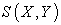of the Levi-Civita connection satisfies the condition(4.1)

Theorem 4.1: An n-dimensional (n > 2) P-Kenmotsu manifold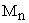is Ricci semisymmetric if and only if it is an Einstein Manifold.

Proof: Let us suppose that a P-Kenmotsu manifold be Ricci semisymmetric. Then from (4.1), we have(4.2)

By puttingin (4.2), we get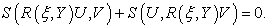(4.3)

Now by using the equations (2.8) (a) and (2.8) (c), the above equation reduces to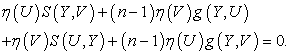(4.4)

Again by puttingin (4.4), we get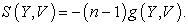(4.5)

This proves that the manifoldis an Einstein manifold.

As an every Einstein manifold is Ricci semisymmetric, the converse of the theorem is trivial.

This completes the proof.

### Statement of Competing Interests

The authors declare that there is no conflict of interests regarding the publication of this paper.

### Acknowledgements

The authors acknowledge Prof. Kalpana of Banaras Hindu University, Varanasi for her valuable suggestions in preparation of the manuscript.

### References

  Sato, I, On a structure similar to the almost contact structure, Tensor (N.S.), 30, 219-224, 1976. In article  Sato, I, On a structure similar to the almost contact structure II, Tensor (N.S.), 31, 199-205, 1977. In article  Adati, T. and Matsumoto, K, On conformally recurrent and conformally symmetric P-Sasakian Manifolds, TRU Math., 13, 25-32, 1977. In article  Adati, T. and Miyazawa, T, On P-Sasakian manifolds admitting some parallel and recurrent tensors, Tensor (N.S.), 33, 287-292, 1979. In article  De, U. C. and Avijit Sarkar, On a type of P-Sasakian manifolds, Math. Reports, 11(2), 139-144, 2009. In article  Matsumoto, K., Ianus, S. and Ion Mihai, On P-Saskian manifolds which admit certain tensor fields, Publ. Math. Debrecen, 33, 61-65, 1986. In article  Kenmotsu, K, A class of almost contact Riemannian manifolds, Tohoku Math. Journal, 24, 93-103, 1972. In article View Article  Sinha, B. B. and Sai Prasad, K. L, A class of almost para contact metric Manifold, Bulletin of the Calcutta Math. Soc., 87, 307-312, 1995. In article  Pokhariyal, G. P. and Mishra, R. S, The curvature tensors and their relativistic significance, Yokohoma Math. J., 18, 105-108, 1970. In article  Pokharial, G. P, Study of a new curvature tensor in a Sasakian manifold, Tensor (N.S.), 36, 222-225, 1982. In article  Sai Prasad, K. L. and Satyanarayana, T, Some curvature properties on a Special paracontact Kenmotsu manifold with respect to Semi-symmetric connection, Turkish Journal of Analysis and Number Theory, 3(4), 94-96, 2015. In article View Article

Published with license by Science and Education Publishing, Copyright © 2018 K. L. Sai Prasad, S. Sunitha Devi and G. V. S. R. DeekshituluThis work is licensed under a Creative Commons Attribution 4.0 International License. To view a copy of this license, visit http://creativecommons.org/licenses/by/4.0/